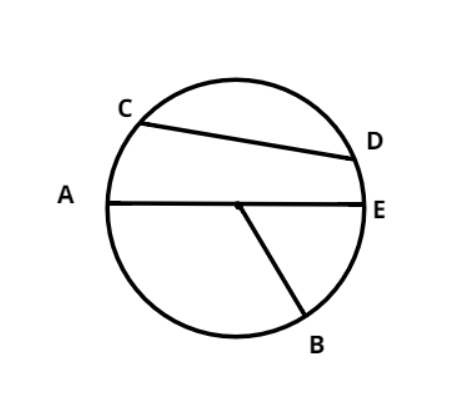Questions & AnswersQuestionAnswers

# The longest chord of a circle is a ………………….. Of the circle.$(a){\text{ }}$Diameter$(b){\text{ }}$Lies on upper part of center$(c){\text{ }}$Lies on lower part of center$(d)$ None of theseAnswer Verified
Hint – A chord is a line connecting any two points within the circle, so try and think of the largest line connecting any two points in terms of length that will give us the answer.Now we have a look at the diagram above then CD is also a chord as it is connecting two points within a circle but however it is not of longest length.
Now let’s take a look at chord AE, it is passing through the center and is largest in length as it is also the diameter of this circle.
Thus the largest chord inside a circle will be a diameter.
Hence correct answer is option (a)

Note – Whenever we face this problem always remember that the diameter is the largest chord within a circle and any line will qualify itself to be chord if and only if it is a line segment having the points of end at the circumference of the circle, thus radius OB can’t be a chord.
Bookmark added to your notes.
View Notes
Radius of a CircleCircumference of a CircleTangent of a CircleEquation of A CircleSecant of a CircleSector of a CircleArea of a CircleArea of a Sector of a Circle FormulaArea of a Circle FormulaConstruction of Tangent to a Circle